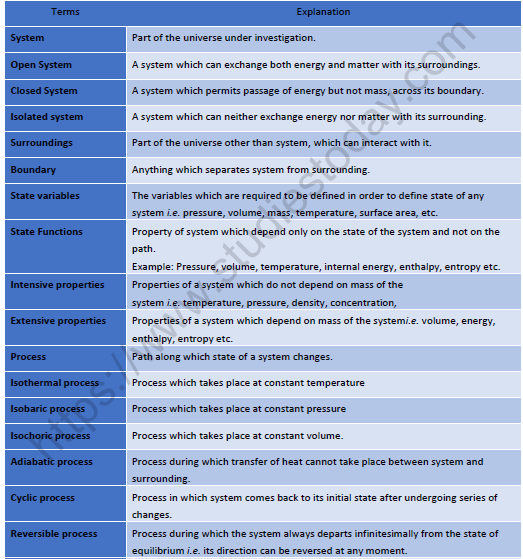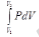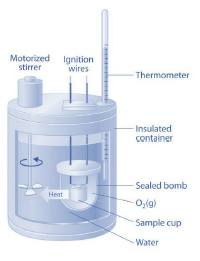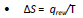# NEET Chemistry Chemical Thermodynamics Revision Notes

Revision Notes on Chemical Thermodynamics

Basic Terminology:Heat, energy and work:

Heat (Q):

Energy is exchanged between system and surround in the form of heat when they are at different temperatures.
Heat added to a system is given by a positive sign, whereas heat extracted from a system is given negative sign.
It is an extensive property.
It is not a state function.

Energy:

It is the capacity for doing work.
Energy is an extensive property.
Unit : Joule.

Work (W):

Work = Force × Displacement i.e. dW = Fdx
Work done on the system is given by positive sigh while work done by the system is given negative sign.
Mechanical Work or Pressure-Volume Work: work associated with change in volume of a system against an external pressure.
Work done in reversible process: W=W = – 2.303 nRT log v2/v1 = –2.303 nRT log p1/p2
Wok done in isothermal reversible contraction of an ideal gas:
?W = – 2.303 nRT log v2/v1 = –2.303 nRT log p1/p2
Unit : Joule.

Internal Energy (E or U):

Sum of all the possible types of energy present in the system.
ΔE = heat change for a reaction taking place at constant temperature and volume.
ΔE is a state function.
It is an extensive property.
Value of ΔE is -ve for exothermic reactions while it is +ve for endothermic reactions.

First Law of Thermodynamics:

Energy can neither be created nor destroyed although it can be converted from one form to another.
or
Energy of an isolated system is constant.

Mathematical Expression

Heat observed by the system = its internal energy + work done by the system.
i.e. q = dE + w

For an infinitesimal process
dq = dE + dw

Where, q is the heat supplied to the system and w is the work done on the system. For an ideal gas undergoing isothermal change ΔE =0.
so q= -w.

For an isolated system, dq=0
so, dE = - dw
For system involving mechanical work only
ΔE = q - pdV
At constant volume i.e. isochoric process
ΔE = qv
For Isothermal Process
ΔE = 0
or
q = - pdV =-W
?q = 0
or
ΔE = W

Enthalpy (H):
H = E+PV
At constant pressure:
dH = dE + pdV

For system involving mechanical work only
dH = QP (At constant pressure)

• For exothermic reactions:
dH = -ve

• For endothermic reactions:
dH = +ve

Relation between dH and dE:

dH = dE + dng RT
Where,
dng = (Number of moles of gaseous products - Number of moles of gaseous reactants)

Heat capacity:

• Amount of heat required to rise temperature of the system by one degree.
C = q / dT

•  Specific heat capacity: Heat required to raise the temperature of 1 g of a substance by one dgree.
Cs = Heat capacity / Mass in grams

•  Molar heat capacity: Heat required to raise the temperature of 1 g of a substance by one dgree.
Cm = Heat capacity / Molar mass.

• Heat capacity of system at constant volume:
Cv = (dE/dT)v

• Heat capacity of system at constant pressure:

Cp = (dE/dT)p
Cp – Cv = R

• Variation Of Heat Of Reaction With Temperature:

dCP = (dH2 - dH1)/(T2-T1) & dCV = (dE2 - dE1)/(T2-T1

• Bomb Calorimeter:Heat exchange = Z × ΔT
Z–Heat capacity of calorimeter system
ΔT– Rise in temp.
Heat changes at constant volumes are expressed in ΔE and Heat changes at constant pressure are expressed in dH.

Enthalpies of Reactions:

Hess’s Law of constant heat summation:

The total enthalpy change of a reaction is the same, regardless of whether the reaction is completed in one step or in several steps.
According to Hess’s law: ΔH = ΔH1 + ΔH2

Born–Haber Cycle:

Applying Hess’s law we get
ΔH1 + 1/2 ΔH2 + ΔH3 + ΔH4 + ΔH5 = ΔHf (MX) (Lattice energy)

Lattice energy: The change in enthalpy that occurs when 1 mole of a solid crystalline substance is formed from its gaseous ions.

Second law of thermodynamics
Statement:
It is impossible to take heat from a hot reservoir and convert it
completely into work by a cyclic process without transferring a part of it to a cold reservoirs.

Mathematically:Where,
ΔS is entropy change.
Entropy is the degree of randomness thus it increases with increase in randomness of particles of the systemi.e. ΔS is positive for melting of ice.
At equilibrium, ΔS = 0
For a spontaneous process, ΔS > 0Gibbs free energy(ΔG):

ΔG = ΔH - TΔS
ΔG = nRT ln Keq
ΔG = nFEcell
At equilibrium, ΔG = 0
For spontaneous process, ΔG < 0

Bond Energies:

Average amount of energy required to break one mole bonds of that type in gaseous molecules.
H–OH(g) → 2H(g) + ½O(g)         ΔH = 498 kJ
O–H(g) → H2(g) + ½O2 (g)        ΔH = 430 Kj
ΔHO–H = (498 + 430)/2 = 464 kJ mol–1

Efficiency of a heat engine (carnot cycle):
W = R (T2 – T1) ln v2/v1
q2 = RT2 ln v2/v1
W = q2## Tags:

Click for more Chemical Thermodynamics Study Material

## Latest NCERT & CBSE News

Read the latest news and announcements from NCERT and CBSE below. Important updates relating to your studies which will help you to keep yourself updated with latest happenings in school level education. Keep yourself updated with all latest news and also read articles from teachers which will help you to improve your studies, increase motivation level and promote faster learning

### Board Cancelled Official CBSE Statement

Keeping in view the requests received from various State Governments and the changed circumstances as on date, following has been decided- 1. Examinations for classes X and XII which were scheduled from 1st July to 15th, 2020 stand cancelled. 2. Assessment of the...

### Board Exams helpline by CBSE

According to the recent announcement of CBSE, the pending Class 10 and 12 Board exams are going to be held from 01 July to 15 July 2020 in India. Along with this, it has also been decided to resume the free of cost tele-counseling service for students from 9.30 am to 5...

### CBSE Reduced Syllabus Class 11 and 12

CBSE has announced major reduction in the syllabus for class 11 and class 12. There has been major changes too in the main syllabus for class 11 and 12. The prevailing health emergency in the country and at different parts of the world as well as the efforts to contain...

### TV Channels for Students by CBSE

In enhancing the students studying part, the Government is planning to introduce one standard one channel plan. The lockdown in India has adversely collapsed all the operations including schools, colleges, and workplaces with unanticipated setbacks. On behalf of the...

### CBSE Online Training for Teachers 2020

It is clear that this pandemic has utterly disrupted an education system that many assert was already losing its relevance. Enhancing professional development digitally is a need in the times of COVID-19, where social distancing and remote interactions is the new...

### CBSE Decision on Board Exam 2020

The Class 10 and 12 exams are cancelled, and results for both 10th and 12th would be declared by July 15 based on internal exams. CBSE Class 12 students would have the option to appear for the exams at a later date. CBSE has a scheme in which marks scored in past 3...

×